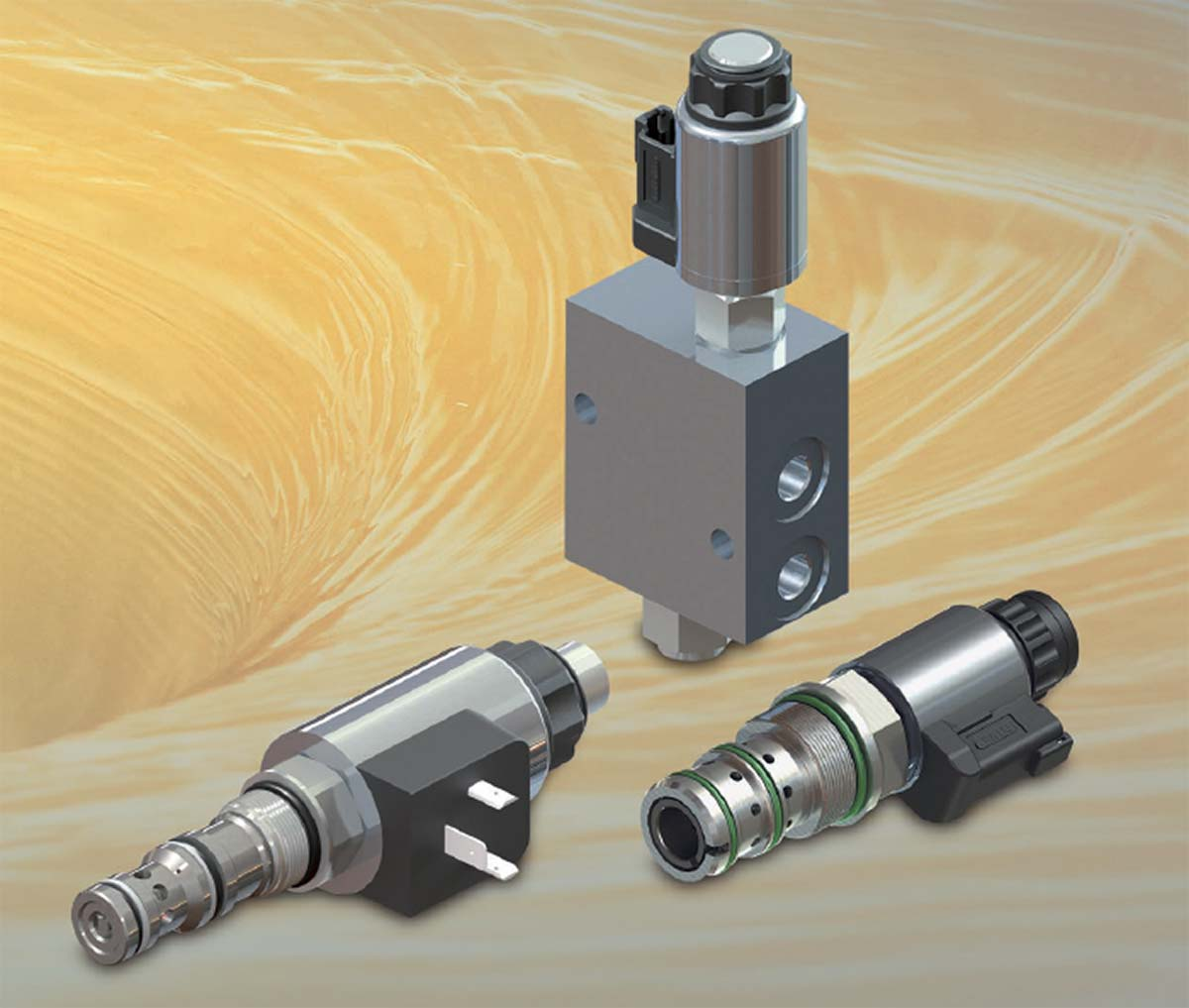# Methods of Flow-Control MeasurementA flow-control valve in engineering can range from a simple opening to a far more complex system. These can include automated closed-loop electrohydraulic valves that detect and react to pressure and temperature variations. At its most basic level, flow control is about regulating the fluid’s speed through the system. The flow-control valve determines the flow rate at any given pressure level.

At Lynch Fluid Control, we have a team of Engineers who specialize in hydraulic systems, including flow control valves and integrating them into full custom manifold solutions. We also support our customers by providing top flow control valve brands off-the-shelf for fast delivery.

## Different Types of Flow Measurement

Controlling the flow of a fluid-power system does not necessarily mean regulating volume per unit of time from a valve. In addition, we can specify the flow rate in three different ways. It is therefore essential to be aware of how flow is to be defined or measured in each case:

Volumetric Flow Rate – Qv

We usually express the volumetric flow rate in units of in .3/sec or min – or cc/sec or cc/min in SI metric measure. This measurement determines the rotational speeds of motor shafts or the linear velocities of piston rods.

To calculate the volumetric flow rate, you need to know the fluid’s velocity and the cross-sectional area of the space through which it is flowing. For example, if a water pipe has a diameter of 10 cm and the water is flowing at a velocity of 2 m/s, the volumetric flow rate would be 20 L/s (litres per second).

Volumetric flow rate is an essential quantity in engineering because we can use it to determine the size of a pipe or other structure needed to transport a given amount of fluid. We can also use it in calculations for pumping systems and heat exchangers.

Weight Flow Rate – Qw

Most often expressed in lb/sec or lb/min units, We can use it to calculate the power using English units of measure.

We often use weight flow rate to calculate the amount of material processed through a system in a given time period. It is also sometimes used to determine the efficiency of a system.

Weight flow rate can be affected by many factors, including the type of material we are processing, the size and shape of the system, and the speed at which the system operates. By understanding the weight flow rate, engineers can design more efficient and effective systems.

Mass Flow Rate – Qg

Typically expressed in slugs/sec or slugs/min units for English measures – or kg/sec or kg/min in SI metric measure – mass flow rate is used to calculate inertia forces during acceleration and deceleration.

Mass flow rate measures the mass of a fluid flowing through a given point in a defined period of time. Mass flow rate is a vital parameter in the design and operation of systems that involve the transport of fluids.

For example, we can use the mass flow rate to calculate the fuel required for a combustion process or the power output of a turbine. We can also use it to determine a pump’s or compressor’s efficiency.